# PSAT Math : Triangles

## Example Questions

← Previous 1 3 4 5 6 7 8 9 14 15

### Example Question #1 : How To Find An Angle In An Acute / Obtuse Isosceles Triangle

Triangle ABC has angle measures as follows: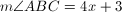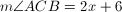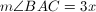What is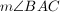?

90

79

57

44

19

57

Explanation:

The sum of the measures of the angles of a triangle is 180.

Thus we set up the equation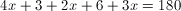After combining like terms and cancelling, we have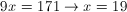Thus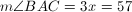### Example Question #1 : How To Find An Angle In An Acute / Obtuse Isosceles Triangle

The base angle of an isosceles triangle is five more than twice the vertex angle.  What is the base angle?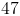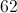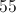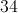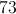Explanation:

Every triangle has 180 degrees.  An isosceles triangle has one vertex angle and two congruent base angles.

Let= the vertex angle and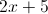= the base angle

So the equation to solve becomes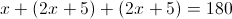Thus the vertex angle is 34 and the base angles are 73.

### Example Question #2 : How To Find An Angle In An Acute / Obtuse Isosceles Triangle

The base angle of an isosceles triangle is 15 less than three times the vertex angle.  What is the vertex angle?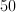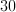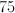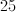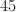Explanation:

Every triangle contains 180 degrees.  An isosceles triangle has one vertex angle and two congruent base angles.

Let= vertex angle and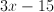= base angle

So the equation to solve becomes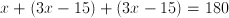.

### Example Question #1 : How To Find An Angle In An Acute / Obtuse Triangle

The base angle of an isosceles triangle is ten less than twice the vertex angle.  What is the vertex angle?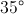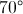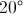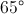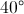Explanation:

Every triangle has 180 degrees.  An isosceles triangle has one vertex angle and two congruent base angles.

Let= vertex angle and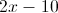= base angle

So the equation to solve becomes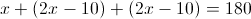So the vertex angle is 40 and the base angles is 70

### Example Question #1 : How To Find An Angle In An Acute / Obtuse Isosceles Triangle

The base angle of an isosceles triangle is 10 more than twice the vertex angle.  What is the vertex angle?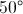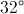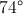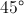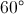Explanation:

Every triangle has 180 degrees.  An isosceles triangle has one vertex angle and two congruent base angles.

Let= the vertex angle and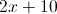= the base angle

So the equation to solve becomes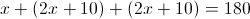The vertex angle is 32 degrees and the base angle is 74 degrees

### Example Question #1 : How To Find An Angle In An Acute / Obtuse Isosceles Triangle

In an isosceles triangle, the vertex angle is 15 less than the base angle.  What is the base angle?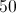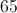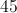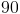Explanation:

Every triangle has 180 degrees.  An isosceles triangle has one vertex angle and two congruent base angles.

Let= base angle and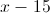= vertex angle

So the equation to solve becomes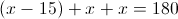Thus, 65 is the base angle and 50 is the vertex angle.

### Example Question #1 : How To Find An Angle In An Acute / Obtuse Isosceles Triangle

In an isosceles triangle the vertex angle is half the base angle.  What is the vertex angle?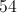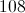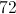Explanation:

Every triangle has 180 degrees.  An isosceles triangle has one vertex angle and two congruent base angles.

Let= base angle and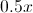= vertex angle

So the equation to solve becomes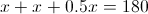, thus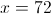is the base angle and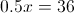is the vertex angle.

### Example Question #1 : How To Find An Angle In An Acute / Obtuse Isosceles Triangle

If the average (arithmetic mean) of two noncongruent angles of an isosceles triangle is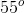, which of the following is the measure of one of the angles of the triangle?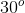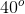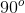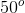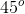Explanation:

Since the triangle is isosceles, we know that 2 of the angles (that sum up to 180) must be equal. The question states that the noncongruent angles average 55°, thus providing us with a system of two equations: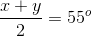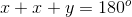Solving for x and y by substitution, we get x = 70° and y = 40° (which average out to 55°).

70 + 70 + 40 equals 180 also checks out.

Since 70° is not an answer choice for us, we know that the 40° must be one of the angles.

### Example Question #9 : How To Find An Angle In An Acute / Obtuse Isosceles Triangle

The base angle of an isosceles triangle is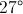.  What is the vertex angle?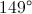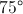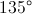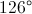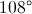Explanation:

Every triangle has 180 degrees.  An isosceles triangle has one vertex angle and two congruent base angles.

Solve the equation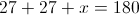for x to find the measure of the vertex angle.

x = 180 - 27 - 27

x = 126

Therefore the measure of the vertex angle is.

### Example Question #1 : Acute / Obtuse Isosceles Triangles

Two sides of an isosceles triangle are 20 and 30. What is the difference of the largest and the smallest possible perimeters?

0

30

15

10